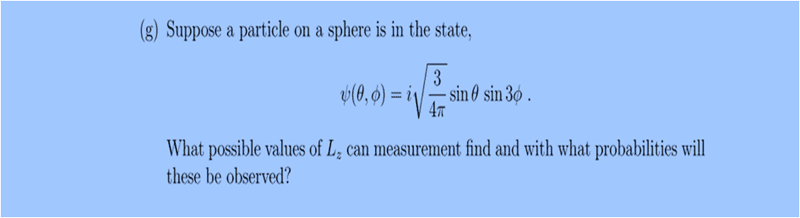# Spherical Harmonics (QM)

1. Homework Statement## Homework Equations

Here we have to express ##\psi(\theta,\phi)## in terms of spherical harmonics ##Y_{lm}## to find the angular momentum.

If ##\psi(\theta,\phi) = i \sqrt{\frac{3}{4\pi}} \sin{\theta} \sin{\phi} ##, it can be written as:
$$\frac{i}{\sqrt{2}} (Y_{1,1}- Y_{1,-1})$$
since :
## Y_{1,\pm1} = \mp \sqrt{\frac{3}{8\pi}} \sin{\theta} e^{\pm i \phi}##,

But now as ##\psi(\theta,\phi)## has ##\sin{3 \phi}## instead of ##\sin{ \phi}##, how will it be represented ?

S.

stevendaryl
Staff Emeritus
First of all, since $L_z = -i \hbar \frac{\partial}{\partial \phi}$, the $\theta$-dependence is irrelevant. So the real question is: how do you write $sin 3\phi$ as a sum of terms of the form $e^{i m \phi}$?

## \sin{3\phi} = \frac{e^{3i\phi } - e^{-3i\phi } }{2i} ## , but alternatively I want to express ## \sin{3\phi} ## in terms of ## \sin{\phi} ## (I guess ), in order to use ##Y_{1,\pm 1}##. Also I tried to find any ##Y_{lm}## defined by ## \sin{m\phi} ## or ## e^{\pm3i\phi } ## but I didn't find, look for example at Table : 5.2 " [Nouredine_Zettili]_Quantum_Mechanics_Concepts ". So any ideas ..

stevendaryl
Staff Emeritus
## \sin{3\phi} = \frac{e^{3i\phi } - e^{-3i\phi } }{2i} ## , but alternatively I want to express ## \sin{3\phi} ## in terms of ## \sin{\phi} ## (I guess ), in order to use ##Y_{1,\pm 1}##. Also I tried to find any ##Y_{lm}## defined by ## \sin{m\phi} ## or ## e^{\pm3i\phi } ## but I didn't find, look for example at Table : 5.2 " [Nouredine_Zettili]_Quantum_Mechanics_Concepts ". So any ideas ..

Why do you want to write it in terms of $Y_{1, \pm 1}$? In general, you write a function $f(\theta, \phi)$ in the form:

$f(\theta, \phi) = \sum_{m l}C_{lm} Y_{lm} (\theta, \phi)$

Since each $Y_{lm} \propto e^{im\phi}$, there are only two terms involved in the sum over $m$: $m=\pm 3$. So your case boils down to:

$sin(\theta) sin(3 \phi) = \sum_l (C_{l, +3} Y_{l, +3} + C_{l, -3} Y_{l, -3})$

You don't actually need to solve for the coefficients $C_{l, \pm 3}$

vela
Staff Emeritus
$$C_{lm} = \int Y^*_{lm}(\theta,\phi) \psi(\theta,\phi)\,d\Omega.$$ Try evaluating the phi integral, and you'll see why ##m=\pm 3## and why the ##\theta## dependence doesn't really matter in this problem.